# Voltmeter Design

## DC Electric Circuits

• #### Question 1

Suppose I were about to measure an unknown voltage with a manual-range voltmeter. This particular voltmeter has several different voltage measurement ranges to choose from:

500 volts
250 volts
100 volts
50 volts
25 volts
10 volts
5 volts

What range would be best to begin with, when first measuring this unknown voltage with the meter? Explain your answer.

• #### Question 2

What would happen to this meter movement, if connected directly to a 6-volt battery?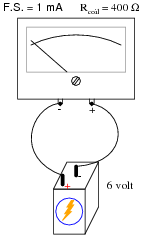• #### Question 3

An important step in building any analog voltmeter or ammeter is to accurately determine the coil resistance of the meter movement. In electrical metrology, it is often easier to obtain extremely precise (“standard”) resistance values than it is to obtain equally precise voltage or current measurements. One technique that may be used to determine the coil resistance of a meter movement without need to accurately measure voltage or current is as follows.

First, connect a decade box type of variable resistance in series with a regulated DC power supply, then to the meter movement to be tested. Adjust the decade box’s resistance so that the meter movement moves to some precise point on its scale, preferably the full-scale (100%) mark. Record the decade box’s resistance setting as R1:Then, connect a known resistance in parallel with the meter movement’s terminals. This resistance will be known as Rs, the shunt resistance. The meter movement deflection will decrease when you do this. Re-adjust the decade box’s resistance until the meter movement deflection returns to its former place. Record the decade box’s resistance setting as R2:The meter movement’s coil resistance (Rcoil) may be calculated following this formula:

 Rcoil = Rs R2 (R1 − R2)

Your task is to show where this formula comes from, deriving it from Ohm’s Law and whatever other equations you may be familiar with for circuit analysis.

Hint: in both cases (decade box set to R1 and set to R2), the voltage across the meter movement’s coil resistance is the same, the current through the meter movement is the same, and the power supply voltage is the same.

• #### Question 4

 Don’t just sit there! Build something!!

Learning to mathematically analyze circuits requires much study and practice. Typically, students practice by working through lots of sample problems and checking their answers against those provided by the textbook or the instructor. While this is good, there is a much better way.

You will learn much more by actually building and analyzing real circuits, letting your test equipment provide the “answers” instead of a book or another person. For successful circuit-building exercises, follow these steps:

1. Carefully measure and record all component values prior to circuit construction.
2. Draw the schematic diagram for the circuit to be analyzed.
3. Carefully build this circuit on a breadboard or other convenient medium.
4. Check the accuracy of the circuit’s construction, following each wire to each connection point, and verifying these elements one-by-one on the diagram.
5. Mathematically analyze the circuit, solving for all values of voltage, current, etc.
6. Carefully measure those quantities, to verify the accuracy of your analysis.
7. If there are any substantial errors (greater than a few percent), carefully check your circuit’s construction against the diagram, then carefully re-calculate the values and re-measure.

Avoid very high and very low resistor values, to avoid measurement errors caused by meter “loading”. I recommend resistors between 1 kΩ and 100 kΩ, unless, of course, the purpose of the circuit is to illustrate the effects of meter loading!

One way you can save time and reduce the possibility of error is to begin with a very simple circuit and incrementally add components to increase its complexity after each analysis, rather than building a whole new circuit for each practice problem. Another time-saving technique is to re-use the same components in a variety of different circuit configurations. This way, you won’t have to measure any component’s value more than once.

• #### Question 5

What is a galvanometer? How might you build your own galvanometer from commonly available components?

• #### Question 6

Describe the design and function of a PMMC style meter movement.

• #### Question 7

We know that connecting a sensitive meter movement directly across the terminals of a substantial voltage source (such as a battery) is a Bad Thing. So, I want you to determine what other component(s) must be connected to the meter movement to limit the current through its coil, so that connecting the circuit to a 6-volt battery results in the meter’s needle moving exactly to the full-scale position:• #### Question 8

Calculate the necessary resistance value and power rating for Rrange in order to make the meter movement respond as a voltmeter with a range of 0 to 100 volts: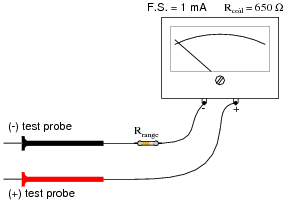• #### Question 9

Calculate the necessary resistance value and power rating for Rrange in order to make the meter movement respond as a voltmeter with a range of 0 to 50 volts: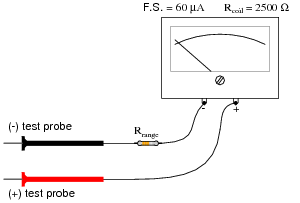• #### Question 10

Calculate the necessary resistance values to give this multi-range voltmeter the ranges indicated by the selector switch positions: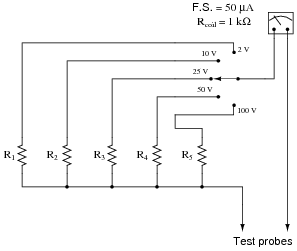• #### Question 11

Calculate the necessary resistance values to give this multi-range voltmeter the ranges indicated by the selector switch positions: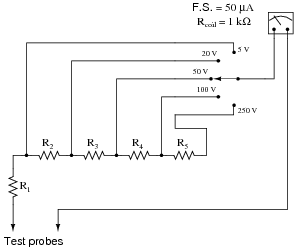• #### Question 12

Ideally, should a voltmeter have a very low input resistance, or a very high input resistance? (Input resistance being the amount of electrical resistance intrinsic to the meter, as measured between its test leads.) Explain your answer.

• #### Question 13

Explain what the ohms-per-volt sensitivity rating of an analog voltmeter means. Many analog voltmeters exhibit a sensitivity of 20 kΩ per volt. Is it better for a voltmeter to have a high ohms-per-volt rating, or a low ohms-per-volt rating? Why?

• #### Question 14

Fundamentally, what single factor in a voltmeter’s design establishes its ohms-per-volt sensitivity rating?

• #### Question 15

Determine the different range values of this multi-range voltmeter: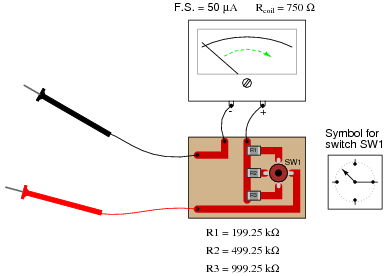All components on the printed circuit board are “surface-mount,” soldered onto the top surfaces of the copper traces. The switch (SW1) schematic diagram is shown to the immediate right of the circuit board, with resistor values shown below the circuit board.

• #### Question 16

What if this voltmeter suddenly stopped working when set in its middle range. The upper and lower ranges still function just fine, though. Identify the most likely source of the problem.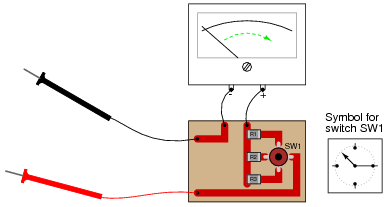• #### Question 17

Suppose you tried to measure the voltage at test point 2 (TP2) with a digital voltmeter having an input resistance of 10 MΩ. How much voltage would it indicate? How much voltage should it ideally indicate?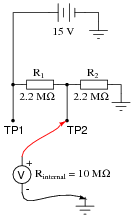• #### Question 18

Suppose you tried to measure the voltage at all three test points with an analog voltmeter having a sensitivity rating of 20 kΩ per volt, set on the 10 volt scale. How much voltage would it indicate at each test point? How much voltage should it ideally indicate at each test point?Test point Ideal voltage Meter indication TP1 TP2 TP3